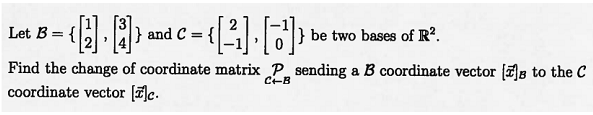# -H3{and C {Let B} be two bases of R2Find the change of coordinate matrix P_ sending a B coordinate vector B to the Ccoordinate vector cC-B

Question
3 viewshelp_outlineImage Transcriptionclose-H 3 { and C { Let B } be two bases of R2 Find the change of coordinate matrix P_ sending a B coordinate vector B to the C coordinate vector c C-B fullscreen
check_circle

Step 1

Required to determine the change of basis matrix from B to C.

Step 2

Work out the coordinates of the basis vectors ...

### Want to see the full answer?

See Solution

#### Want to see this answer and more?

Solutions are written by subject experts who are available 24/7. Questions are typically answered within 1 hour.*

See Solution
*Response times may vary by subject and question.
Tagged in

### Math